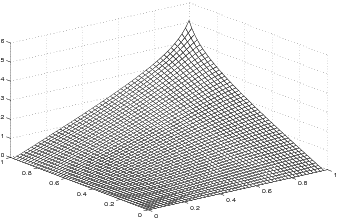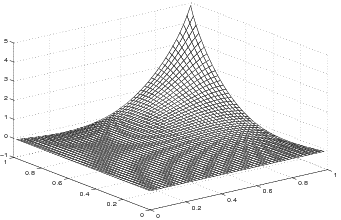]> 2.8 Solution of the Lyapunov equation

### 2.8 Solution of the Lyapunov equation

To ﬁnd the kernel $h$ of the integral operator (2.43), we put $x\left(\theta \right)=\delta \left(\theta -a\right)$, $v\left(\theta \right)=\delta \left(\theta -b\right)$, with $0\le a,b\le 1$, where $\delta$ is the Dirac pseudofunction, in (2.45), which yields
$\begin{array}{cc}\hfill h\left(a,b\right)=h\left(b,a\right)=& \sum _{n=-\infty }^{\infty }{Res}_{\lambda ={\lambda }_{n}}\frac{sinh\sqrt{-\lambda }a}{\sqrt{-\lambda }\left(cosh\sqrt{-\lambda }-K\right)}\frac{sinh\sqrt{\lambda }b}{\sqrt{\lambda }\left(cosh\sqrt{\lambda }-K\right)}=\hfill \\ \hfill =& \sum _{n=-\infty }^{\infty }\frac{2sin{\sqrt{\lambda }}_{n}asinh\sqrt{{\lambda }_{n}}b}{\sqrt{-{\lambda }_{n}}sinh\sqrt{{\lambda }_{n}}\left(cos\sqrt{{\lambda }_{n}}-K\right)},\phantom{\rule{2em}{0ex}}0\le a,b\le 1,\hfill \end{array}$

where $\sqrt{{\lambda }_{n}}={\mu }_{n}$ ($n\in \mathbb{ℤ}$) are given by (2.35). In particular,

$h\left(a,b\right)=\frac{2}{\sqrt{1-{K}^{2}}}\sum _{n=-\infty }^{\infty }\frac{sin\left[a\left(\phi -2n\pi \right)\right]sinh\left[b\left(\phi -2n\pi \right)\right]}{\left(\phi -2n\pi \right)\left[cosh\left(\phi -2n\pi \right)-cos\left(\phi -2n\pi \right)\right]},$

where $\left|K\right|<1$ and $\phi =arccosK$. Employing the partial–fraction expansion of the meromorphic function $z↦\left(sinazsinhbz\right)∕\left(z\left[coshz-cosz\right]\right)$ [56, Problem 5.2.1], we obtain

 $\begin{array}{cc}\hfill h\left(a,b\right)=& \frac{2}{\sqrt{1-{K}^{2}}}\left(\sum _{n=-\infty }^{\infty }\frac{ab}{\phi -2n\pi }+\sum _{k=1}^{\infty }\sum _{n=-\infty }^{\infty }\frac{{B}_{k}}{\phi -2n\pi -{z}_{k}}+\right\hfill \\ \hfill & +\sum _{k=1}^{\infty }\sum _{n=-\infty }^{\infty }\frac{\overline{{B}_{k}}}{\phi -2n\pi +\overline{{z}_{k}}}+\sum _{k=1}^{\infty }\sum _{n=-\infty }^{\infty }\frac{{B}_{k}}{\phi -2n\pi +{z}_{k}}+\hfill \\ \hfill & +\sum _{k=1}^{\infty }\sum _{n=-\infty }^{\infty }\frac{\overline{{B}_{k}}}{\phi -2n\pi -\overline{{z}_{k}}})\hfill \end{array}$ (2.54)

where ${B}_{k}=\left(sina{z}_{k}sinhb{z}_{k}\right)∕\left(sinh{z}_{k}+sin{z}_{k}\right)$ and ${z}_{k}=\left(1+j\right)k\pi$ ($k\in \mathbb{ℕ}$). Taking into account the identity [56, Problem 5.2.4]

$\sum _{n=-\infty }^{\infty }\frac{1}{\phi -2n\pi }=\frac{1}{2}cot\frac{\phi }{2}$

and eliminating $\phi$ in (2.54), and after some transformations, we obtain the ﬁnal formula for $h$, valid for all $K\in \left(-cosh\pi ,1\right)$:

 $\begin{array}{c}h\left(a,b\right)=\frac{ab}{1-K}+\hfill \\ +\frac{1}{\pi }\sum _{k=1}^{\infty }\frac{cos\left(a-b\right)k\pi cosh\left(a+b\right)k\pi -cosh\left(a-b\right)k\pi cos\left(a+b\right)k\pi }{ksinhk\pi \left[coshk\pi -{\left(-1\right)}^{k}K\right]}=\hfill \\ =\frac{ab}{1-K}+\frac{2}{\pi }\sum _{k=1}^{\infty }\left\{\frac{\left[cos\left(a-b\right)k\pi \right]\left[{e}^{\left(a+b-2\right)k\pi }+{e}^{-\left(a+b+2\right)k\pi }\right]}{k\left(1-{e}^{-2k\pi }\right)\left[1+{e}^{-2k\pi }-2K{\left(-1\right)}^{k}{e}^{-k\pi }\right]}-\right\\hfill \\ -\frac{\left[cos\left(a+b\right)k\pi \right]\left[{e}^{\left(a-b-2\right)k\pi }+{e}^{-\left(a-b+2\right)k\pi }\right]}{k\left(1-{e}^{-2k\pi }\right)\left[1+{e}^{-2k\pi }-2K{\left(-1\right)}^{k}{e}^{-k\pi }\right]}}\phantom{\rule{2em}{0ex}}0\le a,b\le 1\hfill \end{array}$ (2.55)

The series in (2.55) converges in square $\left|a\right|+\left|b\right|<2$. From the divergence of the harmonic series and the estimate

$\frac{sinhk\pi }{coshk\pi -{\left(-1\right)}^{k}K}\ge \frac{sinh\pi }{cosh\pi +\left|K\right|},\phantom{\rule{2em}{0ex}}k\in \mathbb{ℕ},$

it follows that the series in (2.55) is divergent at $a=b=1$. Hence, the kernel $h$ is unbounded: $h\left(1,1\right)=\infty$. The plots of the kernel $h$ for two admissible values of $K$ are depicted in Figures 2.5 and 2.6.Figure 2.5: Plot of the kernel $h=h\left(a,b\right)$ for $K=0.75$Figure 2.6: Plot of the kernel $h=h\left(a,b\right)$ for $K=-10$

Numerical calculations show that the folding of $h$ increases for $K$ decreasing from $0$ to $-cosh\pi$. For $K<-1$ the kernel $h$ may take negative values.

Remark 2.8.1. Using (2.25) and (2.43), it is possible to characterize the kernel $h$ by the integral equation

${\int }_{0}^{1}\left[k\left(\tau ,\vartheta \right)h\left(\vartheta ,\theta \right)+h\left(\vartheta ,\tau \right)k\left(\tau ,\theta \right)\right]d\tau =-d\left(\theta \right)d\left(\vartheta \right),\phantom{\rule{2em}{0ex}}0\le \theta ,\vartheta \le 1,$

here, $k$ denotes the kernel of ${\mathcal{𝒜}}^{-1}$ (see (2.31)), and $d$ is deﬁned by (2.8). An approximate solution of this equation can be found with the aid of the inﬂation technique  which allows us to pass to the matrix Lyapunov equation.